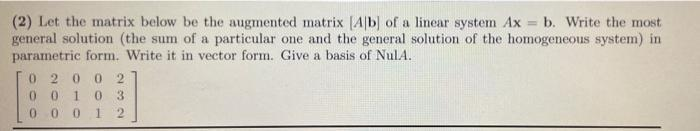Question:

# Let the matrix below be the augmented matrix [A|B] of a linear system Ax = b. Write the most general solution (the sum of a particular one and the general solution of the homogeneous system) in parameLet the matrix below be the augmented matrix [A|B] of a linear system Ax = b. Write the most general solution (the sum of a particular one and the general solution of the homogeneous system) in parametric form. Write it in vector form. Give a basis of NulA. 0 2 0 0 2 0 0 1 0 3 0 0 0 1 2